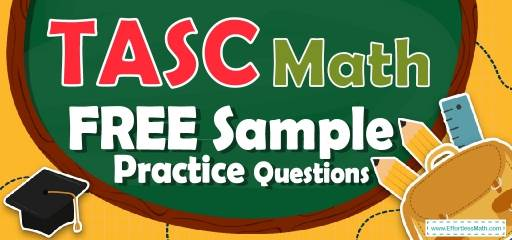# TASC Math FREE Sample Practice QuestionsPreparing for the TASC Math test? To do your best on the TASC Math test, you need to review and practice real TASC Math questions.  There’s nothing like working on TASC Math sample questions to hone your math skills and put you more at ease when taking the TASC Math test. The sample math questions you’ll find here are brief samples designed to give you the insights you need to be as prepared as possible for your TASC Math test.

Check out our sample TASC Math practice questions to find out what areas you need to practice more before taking the TASC Math test!

Start preparing for the 2022 TASC Math test with our free sample practice questions. Also, make sure to follow some of the related links at the bottom of this post to get a better idea of what kind of mathematics questions you need to practice.

## 10 Sample TASC Math Practice Questions

1- Which of the following points lies on the line $$3x+2y=11$$?

A. $$(-1,3)$$

B. $$(2,3)$$

C. $$(-1,7)$$

D. $$(0,2)$$

2- Two-thirds of $$9$$ is equal to $$\frac{2}{5}$$ of what number?

A. 5

B. 9

C. 15

D. 25

3- The marked price of a computer is D dollar. Its price decreased by $$15\%$$ in January and later increased by $$10\%$$ in February. What is the final price of the computer in D dollars?

A. 0.80D

B. 0.93D

C. 0.97D

D. 1.20D

4- A $$45$$ shirt now selling for $$28$$ is discounted by what percent?

A. $$20\%$$

B. $$37.7\%$$

C. $$40.5\%$$

D. $$60\%$$

5- Which of the following could be the product of two consecutive prime numbers?

A. 2

B. 10

C. 24

D. 35

6- Which of the following lists shows the fractions in order from least to greatest?
$$\frac{5}{7},\frac{1}{7},\frac{3}{8},\frac{5}{11}$$

A. $$\frac{3}{8},\frac{1}{7},\frac{5}{7},\frac{5}{11}$$

B. $$\frac{1}{7},\frac{5}{11},\frac{3}{8},\frac{5}{7}$$

C. $$\frac{1}{7},\frac{3}{8},\frac{5}{11},\frac{5}{7}$$

D. $$\frac{3}{8},\frac{1}{7},\frac{5}{11},\frac{5}{7}$$

7- A boat sails $$120$$ miles south and then $$50$$ miles east. How far is the boat from its start point?

A. 45 miles

B. 130 miles

C. 160 miles

D. 170 miles

8- The ratio of boys and girls in a class is $$4:7$$. If there are $$55$$ students in the class, how many more boys should be enrolled to make the ratio $$1:1$$?

A. 8

B. 10

C. 15

D. 20

9- Sophia purchased a sofa for $$530.20$$. The sofa is regularly priced at $$631$$. What was the percent discount Sophia received on the sofa?

A. $$12\%$$

B. $$16\%$$

C. $$20\%$$

D. $$25\%$$

10- The score of Emma was half as that of Ava and the score of Mia was twice that of Ava. If the score of Mia was $$40$$, what is the score of Emma?

A. 5

B. 10

C. 20

D. 40

## Best TASC Math Prep Resource for 2022

1- C
$$3x+2y=11$$. Plug in the values of $$x$$ and $$y$$ from choices provided. Then:
☐A. $$(-1,3) 3x+2y=11→3(-1)+2(3)=11→-3+6=11$$ NOT true!
☐B. $$(2,3) 3x+2y=11→3(2)+2(3)=11→6+6=11$$ NOT true!
☐C. $$(-1,7) 3x+2y=11→3(-1)+2(7)=11→-3+14=11$$ Bingo!
☐D. $$(0,2) 3x+2y=11→3(0)+2(2)=11→0+4=11$$ Nope!

2- C
Let $$x$$ be the number. Write the equation and solve for $$x$$.
$$\frac{2}{3}×9= \frac{2}{5 } . x ⇒ \frac{2×9}{3}= \frac{2x}{5}$$ , use cross multiplication to solve for $$x$$.
$$5×18=2x×3 ⇒90=6x ⇒ x=15$$

3- B
To find the discount, multiply the number by $$(100\%-$$rate of discount).
Therefore, for the first discount we get: $$(D) (100\%-15\%) = (D) (0.85) = 0.85 D$$
For increase of $$10\%: (0.85 D) (100\%+10\%)=(0.85 D) (1.10)=0.93D =93\%$$ of D

4- B
Use the formula for Percent of Change $$\frac{New \ Value-Old \ Value}{Old \ Value}×100\%$$
$$\frac{28-45}{45}×100\%=-37.7\%$$ (Negative sign here means that the new price is less than the old price).

5- D
Some of prime numbers are: $$2,3,5,7,11,13$$. Find the product of two consecutive prime numbers: $$2×3=6$$ (not in the options),$$3×5=15$$ (not in the options),$$5×7=35$$ (bingo!),
$$7×11=77$$ (not in the options)

6- C
Let’s compare each fraction:$$\frac{1}{7} < \frac{3}{8} < \frac{5}{11} < \frac{5}{7}$$. Only choice C provides the right order.

7- B
Use the information provided in the question to draw the shape.
Use Pythagorean Theorem: $$a^2+ b^2=c^2$$
$$120^2+50^2=c^2 ⇒ 14400+2500= c^2 ⇒ 16900=c^2 ⇒ c=130$$
$$\img{https://appmanager.effortlessmath.com/public/images/questions/trteett.png }$$

8- C
The ratio of boys to girls is $$4:7$$. Therefore, there are $$4$$ boys out of $$11$$ students. To find the answer, first, divide the total number of students by $$11$$, then multiply the result by $$4. 55÷11=5 ⇒ 4×5=20$$. There are $$20$$ boys and $$35 (55-20)$$girls. So, $$15$$ more boys should be enrolled to make the ratio $$1:1$$

9- B
The question is this: $$530.20$$ is what percent of $$631$$? Use percent formula:
part$$=\frac{percent}{100}×$$whole. $$530.20=\frac{percent}{100}×631 ⇒ 530.20= \frac{percent ×631}{100} ⇒ 53020 =$$percent $$×631 ⇒$$ percent$$=\frac{53020}{631}=84.02≅84$$ .
$$530.20$$ is $$84\%$$ of $$631$$. Therefore, the discount is: $$100\%-84\%=16\%$$

10- B
If the score of Mia was $$40$$, therefore the score of Ava is $$20$$. Since the score of Emma was half as that of Ava, therefore, the score of Emma is $$10$$.

Looking for the best resource to help you succeed on the TASC Math test?

## More from Effortless Math for TASC Test …

Have a look at our comprehensive TASC Math Worksheets to help you practice all TASC Math concepts and prepare for the TASC Math test.

### Want to review the most common TASC Math formulas?

Here is our complete list of TASC Math formulas.

Have a look at our Full-Length TASC Math Practice Test and Free TASC Math Practice Test.

## Have any questions about the TASC Test?

### What people say about "TASC Math FREE Sample Practice Questions - Effortless Math: We Help Students Learn to LOVE Mathematics"?

No one replied yet.

X
52% OFF

Limited time only!

Save Over 52%

SAVE $40 It was$76.99 now it is \$36.99